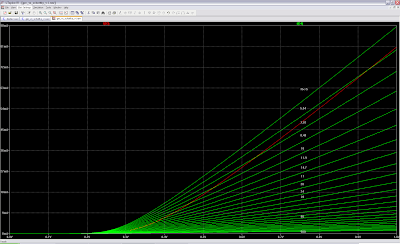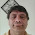## Sunday, 26 January 2014

### Germanium diodes vs Schottky diodes for audio distortion

Germanium diodes are a preferred choice for use in distortion guitar pedals for their unique sounding characteristics when clipping an audio signal. Germanium clipping is softer than that of regular rectifier silicon diodes. A softer clipping generates less high frequency harmonics and hence it produces a more agreeable sound to the ears, closer to that produced by valves.

Germanium diodes also have a lower forward voltage than silicon diodes and hence they are able to clip signals at lower levels than silicon diodes.

But unfortunately, germanium diodes are scarce an expensive these days since their use as rectifiers in electronics is quite reduced and they have been displaced by a variety of different types of diodes made out of silicon. Regular silicon diode rectifiers have an abrupt I-V curve more adequate for rectification purposes, which translates in a hard clipping of the signal at approximately 600mV. On the other hand, Germanium diodes have a much less abrupt I-V curve which means that they provide a much softer clipping that starts at approximately 300mV.

Germanium diodes (like 1N34A) are hard to find in usual large distributors such as Digikey, Mouser, Newark, Farnell or RS, they are mostly found in specialised audio and guitar pedal boutiques since their use is more and more reduced to audio distortion in fuzz pedals. They are only found in conventional through-hole mounting (glass DO-7 package) but not in SMD packages.
The 1N34A can be found from 0.4€ to 1.71\$ in a glass DO-7 package.

Schottky diodes (like BAT54) are a special but quite common type of silicon diodes used as rectifiers with very low forward voltage 200mV, similar to that of germanium diodes, but they also show a very abrupt I-V curve that generates hard clipping at a much lower voltage than regular silicon diodes. They can be found in many different packages including small SMD packages with the advantage that two diodes can be included in the same package, reducing BoM costs additionally. The BAT54S can be found as cheap as 0.022\$ in SOT-23 SMD package.

In order to be able to replace Germanium diodes by Schottky diodes and reduce the steep ramp it only requires adding a resistor in series with the diode so that an increasing voltage drop is added with an increasing current. Using a variable resistor o potentiometer can additionally provide control on the clipping softness, higher resistor value means more clipping softness.

The figure below shows the schematics used with LTSpice simulator in order to compare the I-V and V-I curves of the most common germanium diode (1N34A) with a quite common and cheap Schottky diode (BAT54).

LTSpice schematic file

The schematics with diodes in series allows comparing voltage drop in both cases: Germanium vs Schottky + series resistor for a given range of current. Simulation is repeated for Schottky diode with different resistor values. Series resistor is entered as parameter Rx from 5 to 500 ohms.

The schematics with diodes in parallel allows comparing current through the diodes in both cases for a given range of input voltage and for different resistor values.

The figure below shows the resulting I-V curves with V ranging from 0V to 1V and current from 0mA to 90mA.The red curve shows the I-V curve of the Germanium diode while green curves show the I-V curve for Schottky diode with different values of series resistor from 5 ohms to 500 ohms, the higher the resistor value the flatter the curve.
As it can be seen, there is no way to exactly replicate the germanium diode curve with a Schottky diode, but the Schottky diode may actually provide softer curves than the germanium diode which is the main purpose of using germanium diodes. For low values of current, the resistor must be higher in order to overlap the germanium curve, when current increases, the resistor must be lower, for 5 ohms both curves run parallel showing an asymptote or convergence in the infinity.

The figure below shows the V-I curves with current ranging from 0mA to 1mA and voltages ranging from 0 to 600mV.At very low current values the voltage curves overlap for highest resistor value (500 ohms), but at higher current values the voltage curves overlap for 67 to 80 ohms.

Let's see the effects of both diodes in a real simulation using soft clipping and hard clipping configurations.
The schematics below show a circuit with both types of diodes using a soft clipping section followed by a hard clipping section.

LTSpice schematic file
Opamp PSpice model library

The soft clipping section consists of an opamp amplifying ten times the input signal. Soft clipping diodes are added in both directions (for positive and negative clipping) in the opamp feedback circuit between the negative input and the output of the opamp. The chosen reference resistor (Rin) between negative opamp input and ground is 10 kohms. The feedback resistor is 100 kohm for a x10 gain of the opamp. Varying this 10k value may require adjusting the series resistor of the Schottky diodes to match germanium diode response. The series resistor value required to match germanium diode response in this case is 250 ohms.

The hard clipping section consists of two diodes in both directions between the output of the opamp and ground after a decoupling capacitor of 4.7uF. The series resistor value required to match germanium diode response in this case is 14 ohms.

Figure below shows soft clipping signal comparison for germanium (green) and Schottky (red) for an input sinusoidal signal of 440Hz and 600mV and series resistor values of 5, 25, 100, 250 and 500 ohms. Soft clipping signal in this simulation is probed between the opamp negative input and the opamp output. As it can be seen, Schottky signal (in red) is in general lower than germanium signal (in green) for most resistor values except for 500 ohms, but the waveform shape seems closer to germanium waveform for 250 ohms.

Figure below shows hard clipping signal comparison for germanium (green) and Schottky (red) for an input sinusoidal signal of 440Hz and 600mV and series resistor values of 5, 10, 15 and 20 ohms. Hard clipping in this simulation is probed between the signal connecting output decoupling cap and diodes, and GND. Schottky signal (in red) is closer to germanium signal (in green) for a series resitor value of 15 ohms.
The figure below shows the time response signal in mV for both soft clipping (top plot) and hard clipping (bottom plot) respectively. The input is a sinusoidal signal of 440 Hz that starts at 1V and exponentially decreases with a time constant of 30 Hz.

The use of a exponentially decreasing signal is chosen to recreate the response to a real audio signal source with different voltage levels from 1V to several tens of mV. Clipping is higher for higher voltage levels. The choice of Schottky and Germanium diodes also allows clipping starting at lower voltage levels (200 to 300 mV) compared to silicon (500 to 600mV) or LED (>1200mV) diodes.

The figure below shows the FFT signal (frequency domain spectrum) in dB between 300 Hz and 30kHz for soft clipping (top plot) and hard clipping (bottom plot) signals respectively using a Blackman window to soften the signals and enhance harmonics visibility.

As it can be seen, frequency spectrum for both types of diodes (germanium and Schottky) are very similar and almost overlap perfectly.
Soft clipping shows a higher level of second order harmonics (nicer to the ears and closer to valve response) which is more suitable for overdrive distortion while hard clipping reinforces third order harmonics (harsher sound) more suitable for fuzz distortion.

### Conclusion

Spice simulations show that germanium diodes can be replaced by Schottky diodes plus series resistor for distortion applications with almost no difference in the waveform signals obtained.
But these simulations must be completed with real life experiments to confirm simulation results.

I plan to implement a version of the famous and noisy Shin-Ei Fuzz-Wah with the option of germanium or Schottky diodes for comparison. Stay tuned to my blog for Spice simulations of this pedal and real implementation of it.

### Notes

The figure below shows the I-V curve at near zero current for Germanium diode (red curve) and Schottky + Rseries (magenta curve). Zero bias resistance is the value of the slope of the previous curve (Rbias=dV/dI) at 0A. Using a function dependent current source (BI in LTSpice) I could simulate a current whose value is the derivative of voltage respect current for the Germanium diode (green curve) and the Schottky + Rseries (cyan curve). The value at 0A is the zero bias resistance, which is 167 Kohm for germanium diode and 257 Kohm for Schottky +Rseries.Zero bias impedance and I-V curve at near zero current

1.Jameco has 1N34As on sale right now (8-1-15)

2.3.Great info, thanks.

4.Q1: In schematic #1, if we have 2 active parts in series, how come the BAT54's curve does not affect the 1n34's curve & vice versa? Q2: Where did you get the model for the 1n34 for LT spice, would love to try this! Q3: Unlike Schottkys, Germaniums have a zero-bias resistance in the 50K range - is this taken into account in the model?

5.In schematic #1 there is a common current and voltage is measured. The voltage between GND and the anode of D2 is compared to the voltage between anode and catode of germanium diode D1. In schematic #2 there is a common voltage and current on D1 is compared to current on D2.

6.I have added a figure at the end of the post showing the zero bias impedance, it is as high as 180K and then it descends quickly to 13K or so. Schottky diode with series resistance is a bit lower at 0.

You can find a Spice model of 1N34A diode here:
http://ltwiki.org/?title=Standard.dio

7.Correction: The Schottky diode + Rseries has a zero bias resistance of 257 Kohm, while germanium diode has a value of 167 Kohm. This zero bias resistance is the slope of the I-V curve at 0A, or the derivative dV/dI at 0A. Using a function dependent current source (BI) I could plot these values.

8.Thanks for this great work

9.The article is much informative which i was searching for .Nice intro good explanation thanks for sharing.
Enrgtech